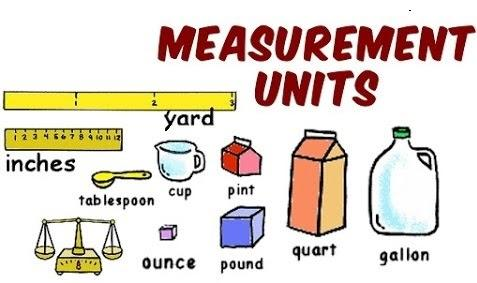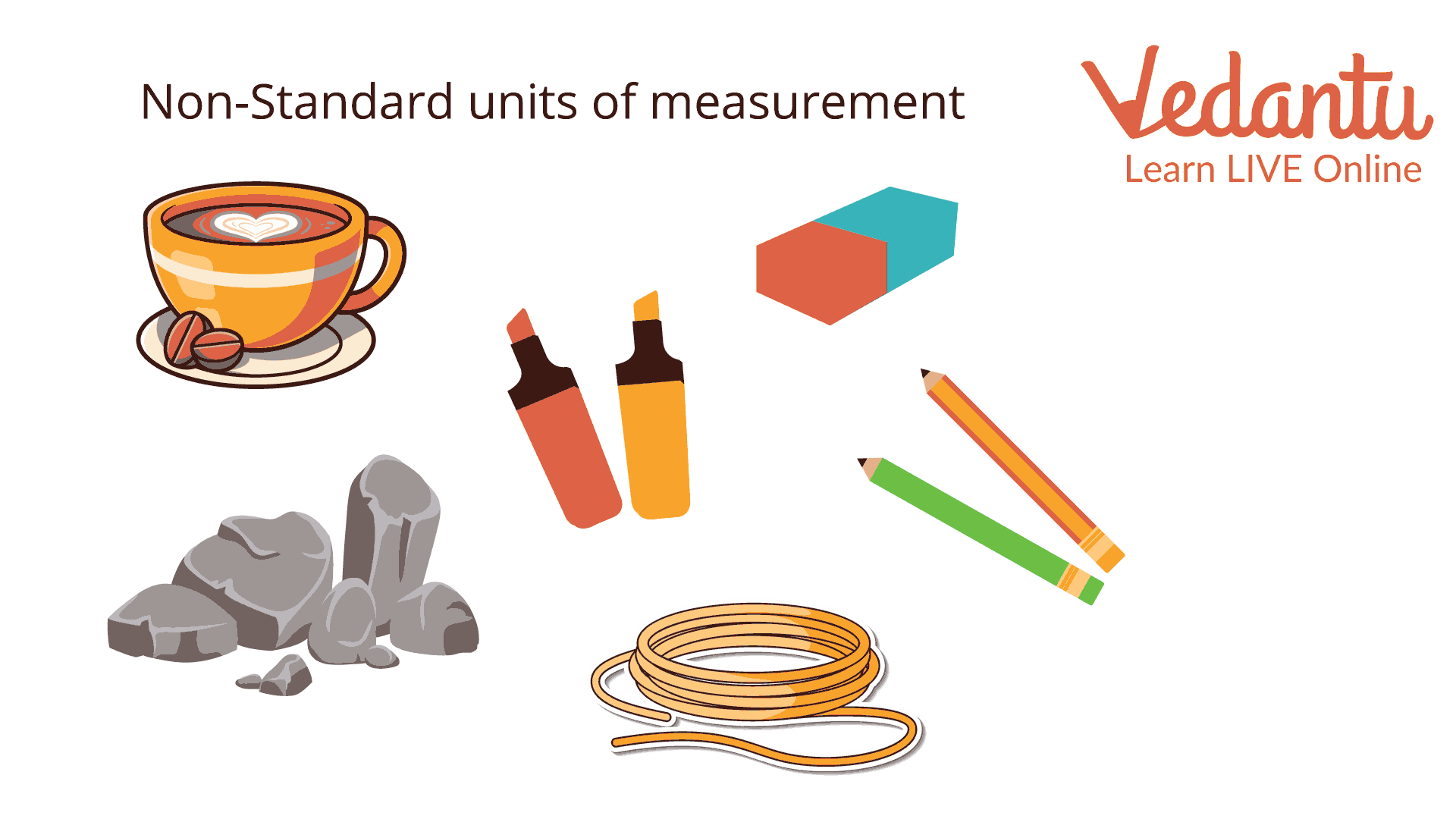Courses
Courses for Kids
Free study material
Offline Centres
More

# Conversion of Units: 1500 MM Into CMLast updated date: 07th Dec 2023
Total views: 101.1k
Views today: 3.01k## Introduction

Kids, have you heard about unit conversion? Conversion of units is the process of changing a quantity's measurement between two different units, which is a multi-step procedure that involves adding, subtracting, or multiplying by a number. There are several techniques to measure weight, distance, and temperature. Temperature is expressed in degrees Celsius, weight is expressed in kilograms, and distance is measured in kilometres.

Let us take an example. In India, we measure temperature in Celsius, while in the US, they do it in Fahrenheit. Here, we need to use unit conversion to convert the temperature in Celsius into Fahrenheit or vice-versa.

## Conversion of Units

Different quantities are measured using various units. Let's examine the measurement systems utilised for the following:

• Temperature

• Distance

• Weight

• Speed

• Area

 Quantity Different Units Length mm, cm, km, miles Temperature Celsius (°C), Fahrenheit (°F), Kelvin (K) Weight g, kg, tonnesMeasurement Units

## Non-Standard Units of Measurement

To measure standard length, we use a ruler, a measuring tape, or a meter stick. We also use invalid nonstandard measurements because they differ from one person to another, such as the hand span, foot span, arm span, pace, and others. Examples of consistent, non-standard length units include blocks, paper clips, and sticks.Non-Standard units of measurement

## SI Units

The seven fundamental quantities of what is now known as the International System of Quantities. These standard units of measurement are decided by the International System of Units (SI), and all derived units can be created by starting with the base units. Like the second for time, the meter for length or distance, and the kilogram for mass are the units and their physical quantities.

 Quantity Name of Unit Abbreviation Length meter m Mass kilogram kg Temperature kelvin K Time second s

## Conversion Table

### Different Units to Measure Length

The figure above shows the length conversion. Moving from left to right, the relationship between the neighbouring units varies by a multiple of 10.

 Unit Converted to SI Unit 1mm (millimetre) 0.001 m 1cm (centimetre) 0.01 m 1dm (decimetre) 0.1 m 1m (metre) 1 m 1dam (decametre) 10 m 1hm (hectometre) 100 m 1km (kilometre) 1000 m 1” (inch) 0.0254 m

### Different Units to Measure Weight

 Unit Converted to SI Unit 1 mg 0.001 g 1 cg 0.01 g 1 dg 0.1 g 1 g 1 g 1 dag 10 g 1 hg 100 g 1 kg 1000 g 1 ton 1000 kg

## Bigger Units to Smaller Units

Question 1:

Convert 4 m to dm.

Solution:

We know 1 m = 10 dm

To convert 4 m to dm, we will need to multiply by 10,

$\begin{array}{c}4 m = \left( {10 \times 4} \right) dm\\ = 40 dm\end{array}$

On converting 4 m to dm, we get 40 dm.

Question 2:

Convert 51 km to m.

Solution:

We know 1 km = 1000 m

In order to convert 50 km to m, we will need to multiply by 1000,

$\begin{array}{c}51 km = \left( {1000 \times 51} \right) m\\ = 51000 m\end{array}$

On converting 51 km to m, we get 51000 m.

Question 3:

Convert 5 kg to g.

Solution:

We know, 1 kg = 1000 g

In order to convert 5 kg to g, we will need to multiply by 1000,

$\begin{array}{c}5 kg = \left( {1000 \times 5} \right) g\\ = 5000 g\end{array}$

On converting 5 kg to g, we get 5000 g.

## Smaller Units to Bigger Units

Question 1:

Convert 90 mm to cm.

Solution:

We know, 10 mm = 1 cm

In order to convert 90 mm to cm, we will need to divide by 10,

$\begin{array}{c}90 mm = \dfrac{{90}}{{10}} cm\\ = 9 cm\end{array}$

On converting 90 mm to cm, we get 9 cm.

Question 2:

Convert 577 cm to dm.

Solution:

We know 1000 cm = 1 dm

In order to convert 577 cm, we will need to divide by 1000,

$\begin{array}{c}577 cm = \dfrac{{577}}{{1000}} dm\\ = 0.577 dm\end{array}$

On converting 577 cm to dm, we get 0.577 dm.

Question 3:

Convert 92 quintals to tonnes.

Solution:

We know 10 quintals = 1 tonne

In order to convert 92 quintals, we will need to divide by 1000,

$\begin{array}{c}92 qu{\mathop{\rm int}} als = \dfrac{{92}}{{1000}} tonnes\\ = 0.092 tonnes\end{array}$

On converting 92 quintals to dm, we get 0.092 tonnes.

### Numbers Nearest to 1000 Conversions

$\begin{array}{l}999 g = 0.999 kg\\999 kg = 0.999{\rm{tonnes}}\\{\rm{999 inches}} = 25.375 m\end{array}$

### Numbers Nearest to 1500 Conversions

$\begin{array}{l}1495 g = 1.495 kg\\1495 miles = 2405.45 km\\1499 yards = 1370.68 m\end{array}$

### Conclusion

A unit conversion is used to express the same property in a different unit of measurement. Like, we know time can be expressed in minutes rather than hours, and distance can be expressed in kilometres, feet, or any other measurement unit instead of miles. To understand better, we convert units of measurement in Mathematics.

## FAQs on Conversion of Units: 1500 MM Into CM

1: Why is unit conversion necessary?

In order to maintain accuracy and problems related to measurement, we must convert between units. For example, we do not measure a pencil's length in kilometres. Therefore, it is necessary to convert kilometres to centimetres.

2. Describe the standard unit.

The value of the standard unit of measurement is a constant that cannot be changed. Measurement must be exact. In the US, the measurement is expressed in feet, inches, and pounds, but in the metric system, it is expressed in meters, centimetres, and kilograms.

3. What kind of measurement does a ruler make?

Length is measured with a ruler. Typically, it is measured in millimetres, centimetres, inches, and feet.

4. What are the fundamental guidelines for unit conversion?

The fundamental rule is to multiply when converting from a larger unit to a smaller unit and divide if it is vice versa.